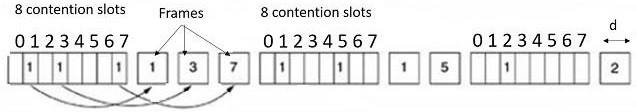# What is Bit Map protocol in computer networks?

Bit map protocol is called collision free Protocol. In bitmap protocol, each contention period consists of exactly N slots. If any station has to send a frame, then it transmits a 1 bit in the respective slot.

## Bitmap Algorithm

The algorithm of Bit Map protocol is explained below −

Step 1 − We use the bitmap or the bit vector which represents a finite set of distinct integers.

Step 2 − To sort the array of integers, initialization of the array size to the specified range is needed and then fill it with zeroes which is a default value in the program followed by setting the corresponding bit in the bitmap to 1 for each integer that was input.

Step 3 − Scanning the bitmap and printing the integers in a sorted order being the final step.

## Explanation

The Bit Map protocol is diagrammatically represented as follows −Here,

Step 1 − Each contention period has exactly N slots. If a station 0 has a frame to send then it transmits 1 bit during slot 0. In general station j may announce that it has a frame to send by inserting 1 bit into slot j.

Step 2 − After all N slots have passed, then each station gets an idea which station is ready to transmit, then the frames are transmitted in numerical order.

Step 3 − Because of mutual understanding there is no chance of collision.

Step 4 − After the last ready station is transmitted its frame, all stations can monitor, another N-bit contention period begins.

Step 5 − If a station becomes ready just after its bit slots have passed by, it must remain silent until the bitmap has come around again.

Step 6 − Protocols like this in which the desire to transmit is broadcast before the actual transmission are called as reservation protocols because they reserve channel ownership in advance and prevent collisions.

## Basic Bitmap: Efficiency

• Channel efficiency/utilization: assume N slots, d bits per frame

Low load: ~ d/(d+N)
High load: ~ d/(d + 1)
• Mean delay

> (N-1)d/2 + N